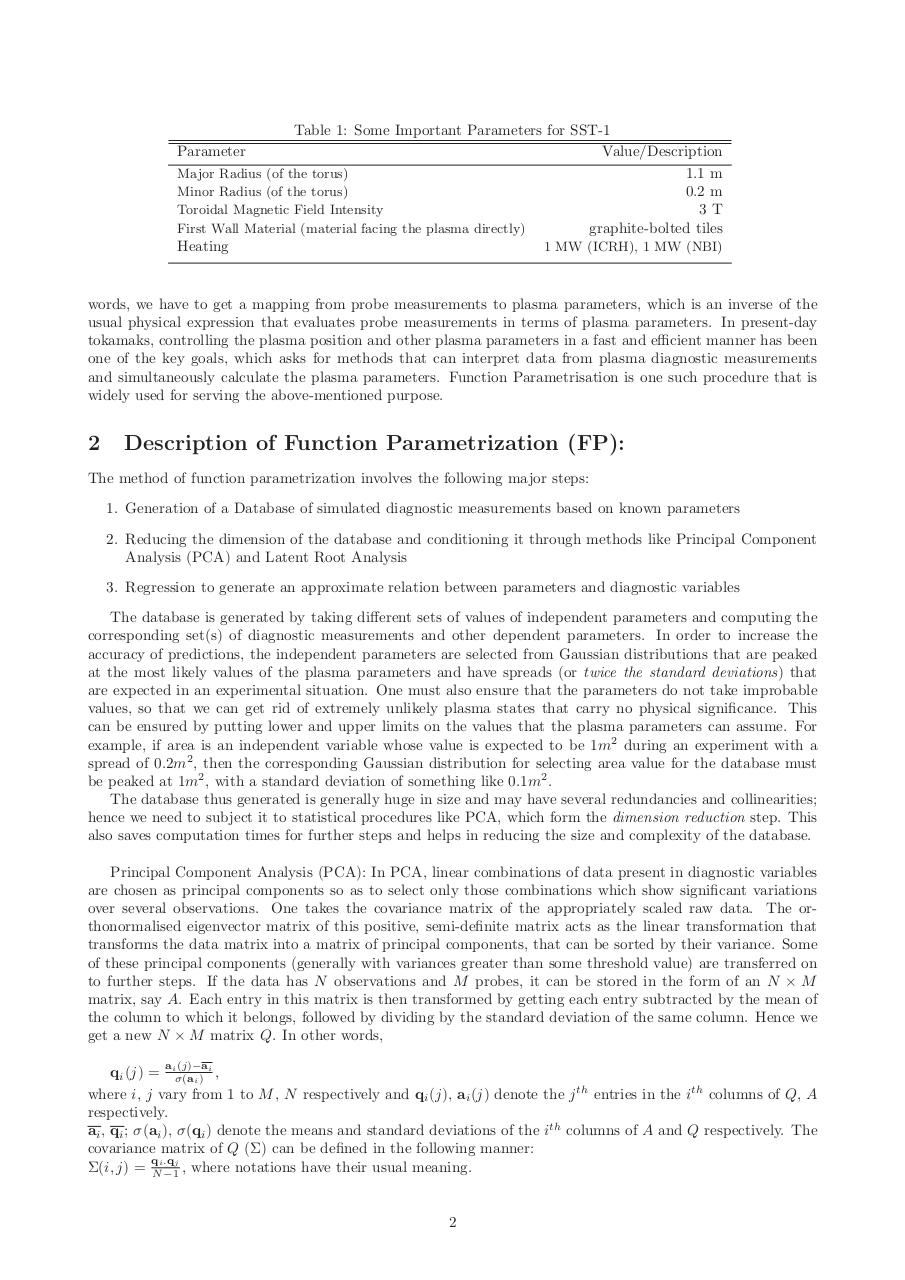# report final.pdfPage 1 2 3 4 5 6 7 8 9 10 11 12

#### Text preview

Table 1: Some Important Parameters for SST-1
Value/Description
1.1 m
0.2 m
Toroidal Magnetic Field Intensity
3T
First Wall Material (material facing the plasma directly)
graphite-bolted tiles
Heating
1 MW (ICRH), 1 MW (NBI)
Parameter

words, we have to get a mapping from probe measurements to plasma parameters, which is an inverse of the
usual physical expression that evaluates probe measurements in terms of plasma parameters. In present-day
tokamaks, controlling the plasma position and other plasma parameters in a fast and efficient manner has been
one of the key goals, which asks for methods that can interpret data from plasma diagnostic measurements
and simultaneously calculate the plasma parameters. Function Parametrisation is one such procedure that is
widely used for serving the above-mentioned purpose.

2

Description of Function Parametrization (FP):

The method of function parametrization involves the following major steps:
1. Generation of a Database of simulated diagnostic measurements based on known parameters
2. Reducing the dimension of the database and conditioning it through methods like Principal Component
Analysis (PCA) and Latent Root Analysis
3. Regression to generate an approximate relation between parameters and diagnostic variables
The database is generated by taking different sets of values of independent parameters and computing the
corresponding set(s) of diagnostic measurements and other dependent parameters. In order to increase the
accuracy of predictions, the independent parameters are selected from Gaussian distributions that are peaked
at the most likely values of the plasma parameters and have spreads (or twice the standard deviations) that
are expected in an experimental situation. One must also ensure that the parameters do not take improbable
values, so that we can get rid of extremely unlikely plasma states that carry no physical significance. This
can be ensured by putting lower and upper limits on the values that the plasma parameters can assume. For
example, if area is an independent variable whose value is expected to be 1m2 during an experiment with a
spread of 0.2m2 , then the corresponding Gaussian distribution for selecting area value for the database must
be peaked at 1m2 , with a standard deviation of something like 0.1m2 .
The database thus generated is generally huge in size and may have several redundancies and collinearities;
hence we need to subject it to statistical procedures like PCA, which form the dimension reduction step. This
also saves computation times for further steps and helps in reducing the size and complexity of the database.
Principal Component Analysis (PCA): In PCA, linear combinations of data present in diagnostic variables
are chosen as principal components so as to select only those combinations which show significant variations
over several observations. One takes the covariance matrix of the appropriately scaled raw data. The orthonormalised eigenvector matrix of this positive, semi-definite matrix acts as the linear transformation that
transforms the data matrix into a matrix of principal components, that can be sorted by their variance. Some
of these principal components (generally with variances greater than some threshold value) are transferred on
to further steps. If the data has N observations and M probes, it can be stored in the form of an N × M
matrix, say A. Each entry in this matrix is then transformed by getting each entry subtracted by the mean of
the column to which it belongs, followed by dividing by the standard deviation of the same column. Hence we
get a new N × M matrix Q. In other words,
(j)−ai
,
qi (j) = aiσ(a
i)
where i, j vary from 1 to M , N respectively and qi (j), ai (j) denote the j th entries in the ith columns of Q, A
respectively.
ai , qi ; σ(ai ), σ(qi ) denote the means and standard deviations of the ith columns of A and Q respectively. The
covariance matrix of Q (Σ) can be defined in the following manner:
q .q
Σ(i, j) = Ni −1j , where notations have their usual meaning.

2